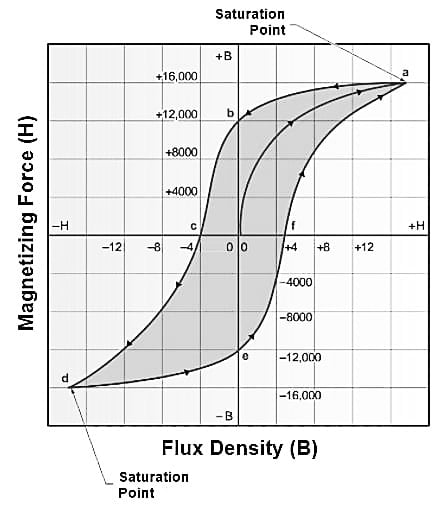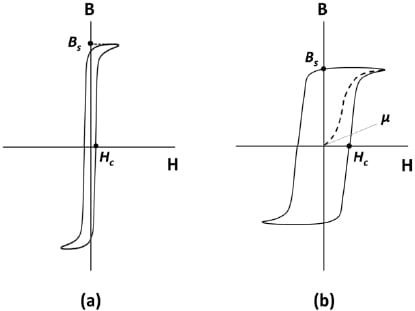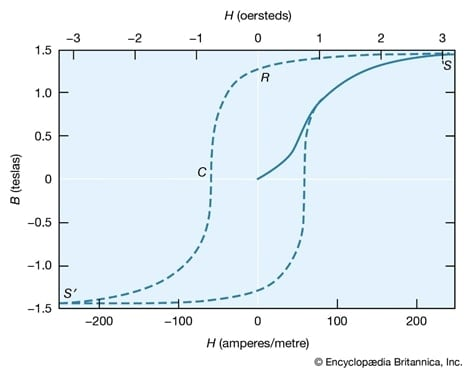Technical Article

# What is a Hysteresis Loop?

September 09, 2021 by Alex Roderick

## Hysteresis is a property of ferromagnetic materials in which a coil's magnetic induction lags the magnetic field that charges the coil.

Each and every molecule of a material is a magnet with a north and a south pole. All molecules must align themselves in the same direction to obtain the maximum flux density. Once all the molecules are aligned, flux density reaches a maximum value, causing the core to become saturated.

The time lag in magnetization of the core behind the value of H (magnetizing force) is caused by molecular agitation. That is, each molecule tends to align itself in the direction of the external field, and then, when the direction of current flow through the coil is reversed, it must align itself in the opposite direction. If the polarity of H is reversed slowly, very little energy is used. If the polarity of H is reversed rapidly, considerable heat is generated by the molecules bumping into each other in the core, resulting in a high-energy loss known as hysteresis loss. The typical hysteresis curve of a ferromagnetic material is shown in Figure 1.##### Figure 1. Typical hysteresis curve of a ferromagnetic material.

With the core completely demagnetized, power is applied. The magnetizing force (H) is increased from the origin (0) to point a, where the core material is saturated at +16,000 G. As magnetizing force is decreased to 0, the core material does not demagnetize at the same rate. At point b with 0 Oe magnetizing force applied, the flux density is still about +12,000 G. At this point, the current through the coil is reversed so that the core magnetizes in the opposite direction. The core is not fully demagnetized until the magnetizing force of 4 Oe is reached at point c. As the magnetization process continues in the negative direction from point c to point d, the polarity of the core reverses and is fully saturated at the magnetizing force of -16,000 G. As the magnetizing force is reduced to 0, the core material still has –12,000 G at point e. Increasing the magnetizing force at this point results in demagnetizing the core at point f and saturating the core in the positive direction at point a.

## What is Retentivity?

Retentivity is the ability of the core material to retain its magnetism after the magnetizing force is removed. Retentivity is most prominent in hard steel and least prominent in soft iron. The value of the residual magnetism when magnetizing force is reduced to 0 depends on the type of core material. Core materials with the greatest retentivity, and therefore the greatest area enclosed by the hysteresis curve, have the greatest energy loss. Materials that have greater hysteresis curve areas typically have greater magnetic retentivity. For example, annealed steel has about one-half the magnetic retentivity as that of hard steel (see Figure 2).## Rectangular Hysteresis Curve

Magnetic substances composed of magnesium, manganese, or iron have a rectangular hysteresis curve. See Figure 3. As the magnetizing force is increased gradually in a positive direction, almost no change in the B occurs. At the point where magnetizing force reaches a critical point (a), the core becomes saturated. As magnetizing force is decreased, the core remains saturated in a positive direction until a critical point (b) is reached, causing the core to saturate in the negative direction. This cycle then repeats.##### Figure 3. Magnetic substances composed of magnesium, manganese, or iron have a rectangular hysteresis curve. Image Courtesy of Britannica

Note

Because the materials are always saturated in a positive or a negative direction, they made excellent devices to store digital information in the 1950s and 1960s, although the memory density was small compared to that of modern technology.

## What is Reluctivity?

Reluctivity is the reluctance per cubic centimeter of material. Reluctivity is sometimes used to compare the reluctance of various materials to air. For example, the air has a reluctivity that is 1800 times that of iron. Reluctivity is the reciprocal of permeability, which is the ability to conduct magnetic lines of force. The larger the reluctivity, the greater the resistance to the magnetic lines of force.

Note

In addition to applications in solenoids, relays, and clutches, electromagnets are used to lift heavy masses of magnetic materials. While permanent magnets have a magnetically retentive (hard) coil material, electromagnets have a non-magnetically retentive (soft) coil material that exhibits magnetic properties only when current flows through the coil. By controlling current flow with a DC power source and a switch, electromagnets can be turned ON and OFF and used to move heavy magnetic materials, such as steel, from one location to another.

## What is Permeance?

Permeance is the property of a core material that allows the passage of magnetic lines of force. Permeance is compared with conductance in an electrical circuit and is the reciprocal of reluctance. Permeance is calculated by applying the following formula:

${{P}_{m}}=\frac{1}{{{R}_{m}}}$

where

Pm = material permanence. The SI unit of magnetic permeance is the henry (H), that is webers per ampere-turn.

Rm = material reluctance in ampere-turns per weber

Example: What is the Permeance of a material with a reluctance of 1.75 × 106 ampere-turns per weber?

${{P}_{m}}=\frac{1}{{{R}_{m}}}=\frac{1}{1.75\text{ }\times {{10}^{6}}}=0.57\text{ }\text{ }\times {{10}^{-6}} \text{ } \text{ } \frac{webers}{ampere-turn}$